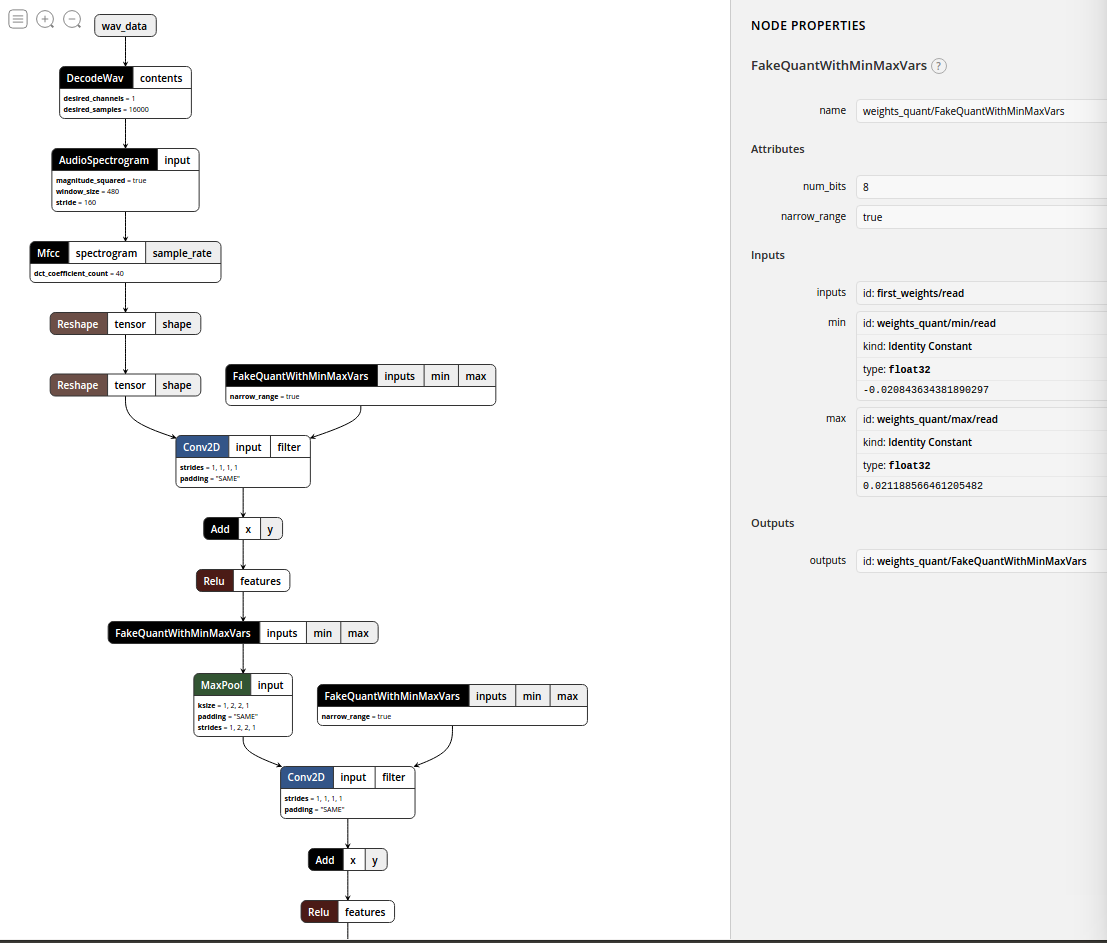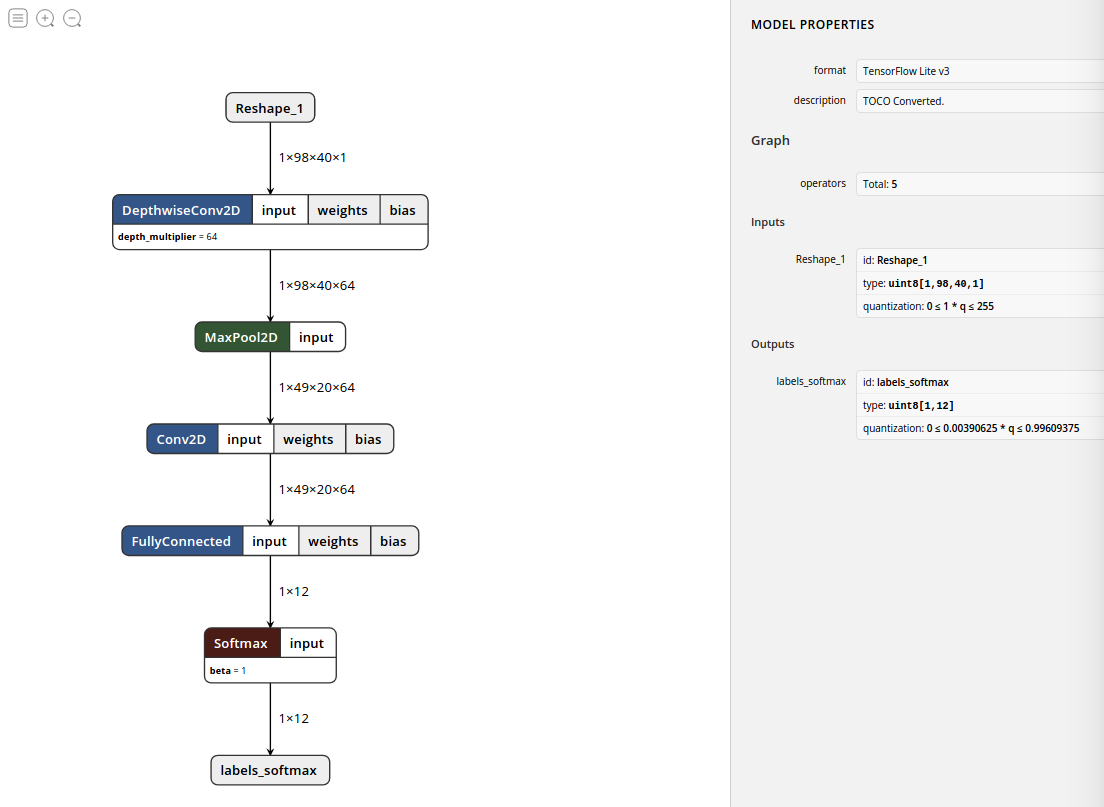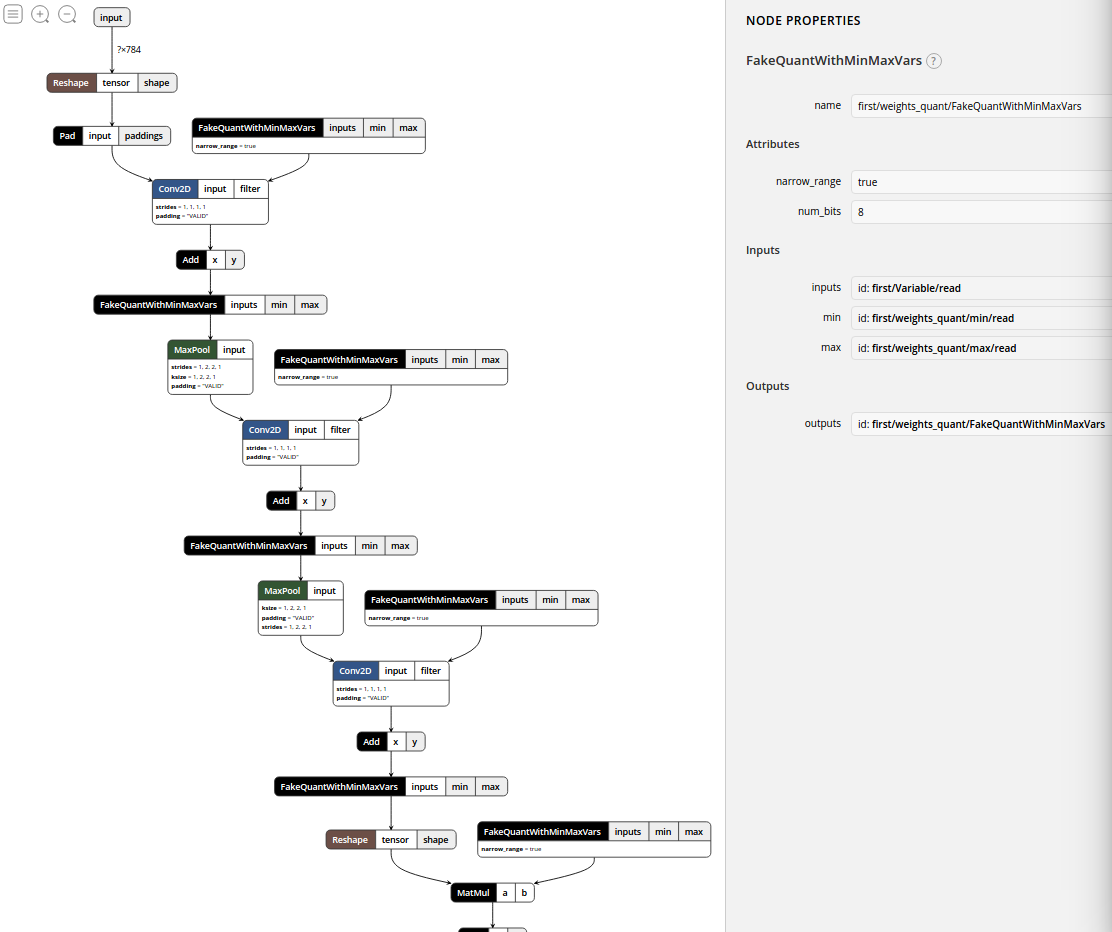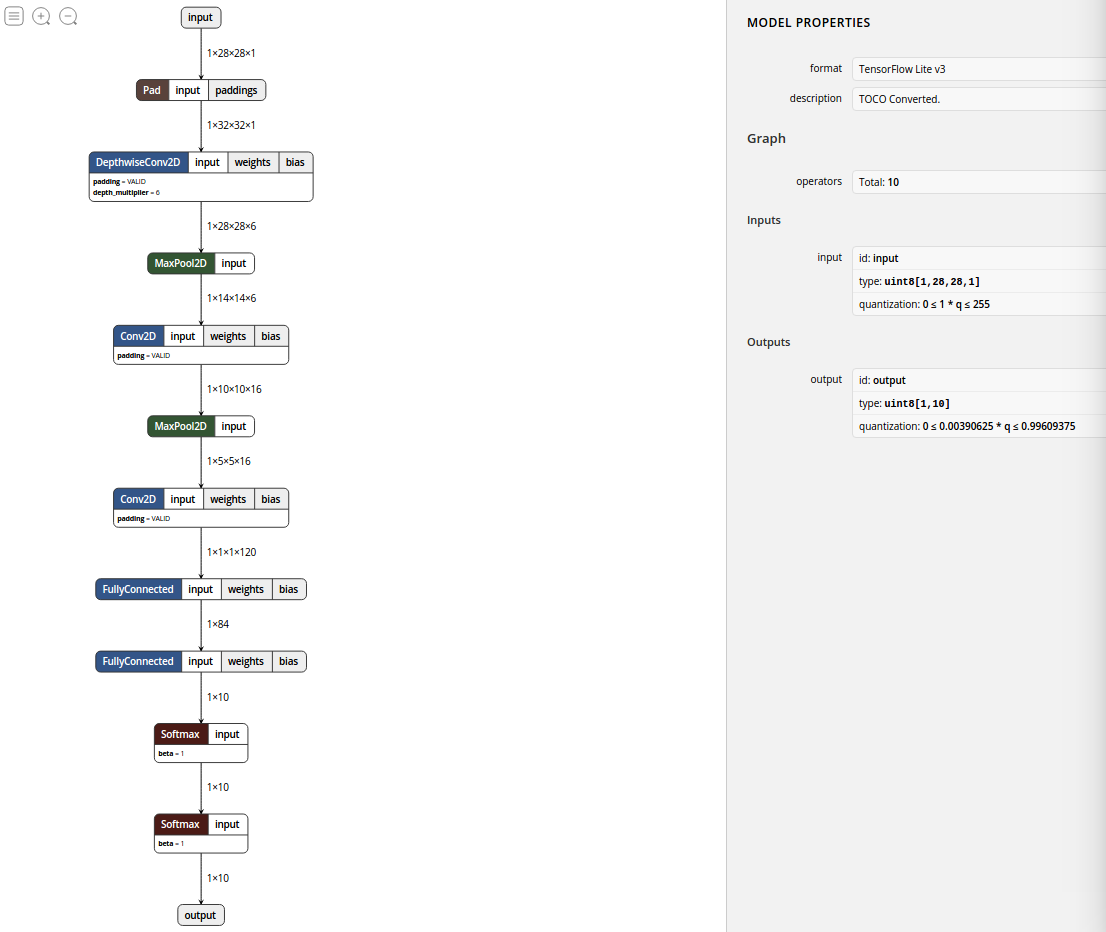# 第二部分：Tensorflow伪量化训练操作（生成量化的tflite）

### 序：

(2018.12.24更新：鉴于评论中有人误解，我这里写清楚一点，后来者也可以先去看看评论区的问题然后再决定要不要看这篇博客～

1.普通量化步骤及使用详见“第一部分”：
2019年2月21日更新：https://blog.csdn.net/angela_12/article/details/84999473
2.伪量化训练，新出现的博客：
[min,max]的部分可以看看：TensorFlow量化训练
3.伪量化含义理解：

### 我的环境：

2018.12.24更新：
1.系统：Ubuntu16.04
2. Python版本：3.5.2
3. TensorFlow Lite(tflite)版本：Release 0.1.7
4. TF版本： 源码安装时版本为1.9.0，后来用pip升级为1.11.0。TF版本查看方法：

import tensorflow as tf
tf.__version__


### 正文：

create_training_graphcreate_eval_graph

##### #############好啦，现在就直接写过程吧##############

1. 在训练图中加入create_training_graph作为fake quantization nodes，然后训练，生成ckpt和pbtxt文件(参考train.py)。
2. 创建推理图(freeze.py里的create_inference_graph())，就是重新调用训练时用的网络模型(例子里就是models.py 的create_model)，然后给出一个输出，也就是logits = models.create_model()和tf.nn.softmax(logits, name=‘labels_softmax’)这两句话，前面的都是数据处理，没什么用。
3. 将上面的输出(训练好的数据)传给create_eval_graph()，就是models.load_variables_from_checkpoint()，这里的eval把这张推理图数据转换成tflite能够识别并量化的格式。
4. 后面的frozen_graph_def把ckpt和pbtxt固化，freeze 成.pb文件，这个图中就是带有fake quantization nodes的了。可以用tensorboard或者Netron来查看图。
5. 上面的就完成了官方文档说的第一步，然后将上面生成的pb文件放到toco工具中，运行就可以生成tflite了。
###### toco工具的安装也是用bazel，编译并执行是下面的指令：

2019.3.6更新： 这里的文件夹换了，改成：tensorflow/lite/toco，注意替换一下！！

bazel run --config=opt tensorflow/contrib/lite/toco:toco -- \
--input_file=/home/.../tensorflow/.../Mnist_train/speech_my_frozen_graph.pb \
--output_file=/home/.../tensorflow/.../Mnist_train/speech_my_frozen_graph.tflite \
--input_format=TENSORFLOW_GRAPHDEF \
--output_format=TFLITE \
--inference_type=QUANTIZED_UINT8 \
--input_shapes=1,98,40,1 \
--input_arrays=Reshape_1 \
--output_arrays=labels_softmax \
--allow_custom_ops

###### 仅执行：
bazel-bin/tensorflow/contrib/lite/toco/toco \
--input_file=/home/.../tensorflow/.../Mnist_train/speech_my_frozen_graph.pb \
--output_file=/home/.../tensorflow/.../Mnist_train/speech_my_frozen_graph.tflite \
--input_format=TENSORFLOW_GRAPHDEF \
--output_format=TFLITE \
--inference_type=QUANTIZED_UINT8 \
--input_shapes=1,98,40,1 \
--input_arrays=Reshape_1 \
--output_arrays=labels_softmax \
--allow_custom_ops


#### 注：

1）tflite文件不只是用在移动端的，PC端也是可以的，感觉是因为移动端主要用的是tflite，然后PC端也可以用Python脚本来使用tflite(用的是tf.contrib.lite.Interpreter)，所以谷歌就都换成tflite了，之前的quantize_graph.py被谷歌删除了，这个工具感觉可以不用了。现在唯一的保证精度的方法就是伪量化训练生成pb，然后toco转化为tflite，toco转化也可以用Python来写，这部分，我之后来补上。
(这一部分，建议参考社区问题Zongjun大神的第一个回答：https://www.tensorflowers.cn/t/7136)
2）将上面input_file(这里的pb文件路径是最后freeze生成的pb路径)和output_file(这里的tflite路径是要生成的tflite要保存的路径)的路径换成自己的路径，写绝对路径比较好。
3）allow_custom_ops:这个指令可以避免一些不必要的错误，允许一些传统方法，相关知识可以去下面的参考博客中去看。
4）–input_shapes它后面那几个要注意末尾的“s”，要么都加，要么都不加。这几个的获取可以用：

sudo bazel-bin/tensorflow/tools/graph_transforms/summarize_graph \
--in_graph=/home/.../tensorflow/Mnist_train/speech_my_frozen_graph.pb


sudo bazel-bin/tensorflow/tools/graph_transforms/summarize_graph \
--in_graph=/home/.../tensorflow/Mnist_train/speech_my_frozen_graph.pb \
--print_structure=true


5）至于有人提到的–default_ranges_min=0 --default_ranges_max=6这两个指令，我觉得它是Post Training Quantization 需要的，这个方法可以不训练直接转化，但是精度不如训练的，应该是差很多，而且还是用float32计算的(这里可能有点问题，需要再想想)。
6）quantization-aware training文章里提到的–std_value=127.5 --mean_value=127.5这两个指令我没用，还没确定这两个怎么用，知道了再来更新。

## 运行speech例子：

2018.12.18更新： 今天tensorflow的官方公众号发布了一篇讲解speech这个例子的文章，感觉挺有用的，只是除了里面的量化没有讲…不过作为了解TF框架的例子来讲还是挺好的，位置在这里。）

1. train .py：没有数据的话，它会自动下载数据包，有2.4G，有语音数据的话，可以自己看看数据格式然后用自己的数据训练。具体可以看train.py上面的注释。要想得到量化的结果记得–quantize这里改成True。
2. freeze.py里面—output_file这里写上自己想要存储的路径。–start_checkpoint这里写上要使用的ckpt文件，格式是：default=’/tmp/speech_commands_train/conv.ckpt-110’。
3. 在Netron中查看的图是这样的：speech.pbspeech.tflite

（2018.12.21补充：speech.pb大小为3.7M，speech.tflite大小为929.5K）

## mnist伪量化训练练习代码：

##### 1.mnist_build_network.py，代码如下：
import tensorflow as tf
# 创建图片占位符：x，标签占位符：y 和 随机失活系数keep_prob，以供处理图片，训练和预测时使用
#[x并不是一个特定的值，它是一个placeholder，一个我们需要输入数值当我们需要tensorflow进行运算时。我们想要输入任意数量的mnist图片，每一个都展开成一个784维的向量。我们用一个二维的[None, 784]浮点张量代表。 (这里的None表示维度可以是任意的长度.)]
x = tf.placeholder("float", shape=[None, 784], name='input')
y = tf.placeholder("float", shape=[None, 10], name='labels')
keep_prob = tf.placeholder("float", name='keep_prob')
# 定义mnist网络结构
def build_network(is_training):
# 定义网络类型
#[我们的模型中也需要权重和bias。我们可以把它们看成是额外的输入，Tensorflow有更加好的方法来表示它: Variable. Variable是一个Tensorflow图交互操作中一个可以修改的张量。 它可以在计算中修改。对于机器学习的，一般都有一些Variable模型参数。]
def weight_variable(shape):
initial = tf.truncated_normal(shape, stddev=0.1)
return tf.Variable(initial)
def bias_variable(shape):
initial = tf.constant(0.1, shape=shape)
return tf.Variable(initial)
# convolution and pooling
def conv2d(x, W):
return tf.nn.conv2d(x, W, strides=[1, 1, 1, 1], padding='VALID')
def max_pool_2x2(x):
return tf.nn.max_pool(x, ksize=[1, 2, 2, 1], strides=[1, 2, 2, 1], padding='VALID')
# convolution layer
def lenet5_layer(layer, weight, bias):
W_conv = weight_variable(weight)
b_conv = bias_variable(bias)
h_conv = conv2d(layer, W_conv) + b_conv
return max_pool_2x2(h_conv)
# connected layer
def dense_layer(layer, weight, bias):
W_fc = weight_variable(weight)
b_fc = bias_variable(bias)
return tf.matmul(layer, W_fc) + b_fc
# 开始搭建网络结构
# first layer
with tf.name_scope('first') as scope:
x_image = tf.pad(tf.reshape(x, [-1,28,28,1]), [[0,0],[2,2],[2,2],[0,0]])
firstlayer = lenet5_layer(x_image, [5,5,1,6], )
# second layer
with tf.name_scope('second') as scope:
secondlayer = lenet5_layer(firstlayer, [5,5,6,16], )
# third layer
with tf.name_scope('third') as scope:
W_conv3 = weight_variable([5,5,16,120])
b_conv3 = bias_variable()
thirdlayerconv = conv2d(secondlayer, W_conv3) + b_conv3
thirdlayer = tf.reshape(thirdlayerconv, [-1,120])
# dense layer1
with tf.name_scope('dense1') as scope:
dense_layer1 = dense_layer(thirdlayer, [120,84], )
# dense layer2
with tf.name_scope('dense2') as scope:
dense_layer2 = dense_layer(dense_layer1, [84,10], )
# 运行得到真实输出：finaloutput
if is_training:
finaloutput = tf.nn.softmax(tf.nn.dropout(dense_layer2, keep_prob), name="softmax")
# 为eval调用准备，eval用的网络要去掉 dropout
else:
finaloutput = tf.nn.softmax(dense_layer2, name='softmax')
print('finaloutput:', finaloutput)
return finaloutput


##### 2. mnist_fakequantize_train.py代码：
import tensorflow as tf
from tensorflow.examples.tutorials.mnist import input_data
from Mnist_train.mnist_build_network import build_network, x, y, keep_prob

# 加载mnist下载的数据，有四个，数据我是在一个博客给出的百度网盘里下的，不过我的git账号还没弄好，不能上传，需要的话可以去看mnist实现的相关博客找数据下载。
mnist = input_data.read_data_sets("MNIST_data/", one_hot=True)

def create_training_graph():
#创建训练图，加入create_training_graph：
g = tf.get_default_graph()   # 给create_training_graph的参数，默认图
#调用网络定义，也就是拿到输出
logits = build_network(is_training=True)    #这里的is_training设置为True，因为前面模型定义写了训练时要用到dropout
# 写loss，mnist的loss是用交叉熵来计算的，loss和optimize方法可以根据自己的情况来设置。
with tf.name_scope('cross_entropy'):
cross_entropy_mean = tf.reduce_mean(tf.nn.softmax_cross_entropy_with_logits_v2(labels=y, logits=logits))
print('cost:', cross_entropy_mean)
# 加入 create_training_graph函数，注意位置要在loss之后， optimize之前
# if FLAGS.quantize:
# 上面这句是如果用parser设置flag参数的话，就用这种方式设置开关，用法可以自己查一下，或者参考speech的例子就知道了。
tf.contrib.quantize.create_training_graph(input_graph=g, quant_delay=0)
#  optimize用原来的Adam效果较好，不知道我这里为什么用GradientDescentOptimizer的话，基本不收敛。
optimize = tf.train.AdamOptimizer(1e-4).minimize(cross_entropy_mean)
# optimize = tf.train.GradientDescentOptimizer(1e-4).minimize(cross_entropy_mean)
# 比较输出类别概率的最大值[tf.argmax 是项的极其有益的函数，它给返回在一个标题里最大值的索引。例如，tf.argmax(y,1) 是我们的模型输出的认为是最有可能是的那个值，而 tf.argmax(y_,1) 是正确的标签的标签。]
prediction_labels = tf.argmax(logits, axis=1, name="output")
# 将得出的最大值与实际分类标签对比，看二者是否一致[如果我们的预测与匹配真正的值，我们可以使用tf.equal来检查。]
correct_prediction = tf.equal(tf.argmax(logits, 1), tf.argmax(y, 1))
# 给出识别准确率[这会返回我们一个布尔值的列表.为了确定哪些部分是正确的，我们要把它转换成浮点值，然后再示均值。 比如, [True, False, True, True] 会转换成 [1,0,1,1] ，从而它的准确率就是0.75.]
accuracy = tf.reduce_mean(tf.cast(correct_prediction, "float"))
#后面with这部分对于量化应该没啥影响，记得是tensorboard要用的，应该是出准确率啥的曲线图的……（不过这里应该没用到吧，，我只是搬过来了还没看，会用的想用就用吧，不会的就删掉吧）
with tf.get_default_graph().name_scope('eval'):
tf.summary.scalar('cross_entropy', cross_entropy_mean)
tf.summary.scalar('accuracy', accuracy)
# 返回所需数据，供训练使用
return dict(
x=x,
y=y,
keep_prob=keep_prob,
optimize=optimize,
cost=cross_entropy_mean,
correct_prediction=correct_prediction,
accuracy=accuracy,
)

#开始训练
def train_network(graph):
# 初始化
init = tf.global_variables_initializer()
# 调用Saver函数保存所需文件
saver = tf.train.Saver()
# 创建上下文，开始训练sess.run(init)
with tf.Session() as sess:
sess.run(init)
# 一共训练两万次，也可以更多，不过两万次感觉准确率就能达到将近1了
for i in range(20000):
# 每次处理50张图片
batch = mnist.train.next_batch(50)
# 每100次保存并打印一次准确率等
if i % 100 == 0:
# feed_dict喂数据
train_accuracy = sess.run([graph['accuracy']], feed_dict={
graph['x']:batch,    # batch存的图片数据
graph['y']:batch,    # batch存的标签
graph['keep_prob']: 1.0})      # 随机失活(全部？)
print("step %d, training accuracy %g"%(i, train_accuracy))
sess.run([graph['optimize']], feed_dict={
graph['x']:batch,
graph['y']:batch,
graph['keep_prob']:0.5})
test_accuracy = sess.run([graph['accuracy']], feed_dict={
graph['x']: mnist.test.images,
graph['y']: mnist.test.labels,
graph['keep_prob']: 1.0})
print("Test accuracy %g" % test_accuracy)
# 保存ckpt(checkpoint)和pbtxt。记得把路径改成自己的路径，写不好相对路径的就直接写绝对路径。绝对路径就是我写的这种完整的路径。
saver.save(sess, '/home/angela/tensorflow/tensorflow/Mnist_train/mnist_fakequantize.ckpt')
tf.train.write_graph(sess.graph_def, '/home/angela/tensorflow/tensorflow/Mnist_train/', 'mnist_fakequantize.pbtxt', True)

def main():
g1 = create_training_graph()
train_network(g1)

main()

##### 3. mnist_fakequantize_freeze.py：代码：
import tensorflow as tf
import os.path
from Mnist_train.mnist_build_network import build_network
from tensorflow.python.framework import graph_util

# 创建推理图
def create_inference_graph():
"""Build the mnist model for evaluation."""
# 调用网络，Create an output to use for inference.
logits = build_network(is_training=False)
# 得到分类输出
tf.nn.softmax(logits, name='output')

def load_variables_from_checkpoint(sess, start_checkpoint):
"""Utility function to centralize checkpoint restoration.
Args:
sess: TensorFlow session.
start_checkpoint: Path to saved checkpoint on disk.
"""
saver = tf.train.Saver(tf.global_variables())
saver.restore(sess, start_checkpoint)

def main():
# Create the model and load its weights.
init = tf.global_variables_initializer()
with tf.Session() as sess:
sess.run(init)
# 推理图
create_inference_graph()
# 加入create_eval_graph()，转化为tflite可接受的格式。以下语句中有路径的，记得改路径。
# if FLAGS.quantize:
tf.contrib.quantize.create_eval_graph()
load_variables_from_checkpoint(sess, '/home/angela/tensorflow/tensorflow/Mnist_train/mnist_fakequantize.ckpt')
# Turn all the variables into inline constants inside the graph and save it.
# 固化 frozen：ckpt + pbtxt
frozen_graph_def = graph_util.convert_variables_to_constants(
sess, sess.graph_def, ['output'])
# 保存最终的pb模型
tf.train.write_graph(
frozen_graph_def,
os.path.dirname('/home/angela/tensorflow/tensorflow/Mnist_train/mnist_frozen_graph.pb'),
os.path.basename('/home/angela/tensorflow/tensorflow/Mnist_train/mnist_frozen_graph.pb'),
as_text=False)
tf.logging.info('Saved frozen graph to %s', '/home/angela/tensorflow/tensorflow/Mnist_train/mnist_frozen_graph.pb')

main()


（2018.12.21补充：mnist.pb大小为256.3K，mnist.tflite大小为66.4K）

##### toco转化为tflite：
bazel-bin/tensorflow/contrib/lite/toco/toco \
--input_file=/home/.../tensorflow/.../Mnist_train/mnist_fakequantize.pb \
--output_file=/home/.../tensorflow/.../Mnist_train/mnist_fakequantize.tflite \
--input_format=TENSORFLOW_GRAPHDEF \
--output_format=TFLITE \
--inference_type=QUANTIZED_UINT8 \
--input_shapes=1,28,28,1 \
--input_arrays=input \
--output_arrays=output \
--allow_custom_ops


#### Netron看图：mnist.pbmnist.tflite

#### 注：

1）图片里的是之前训练和转化得出来的结果，但是现在里面的input都没有了，我也不知道为什么，现在的指令都是按照官方文档写的，出来后是没有input的，但是之前是有的，然后我测试没有input的结果是正确的，所以不知道这有什么影响，可能之前用的指令在inference_type那里不太一样吧。这个我有时间再研究一下，也可能是我TF版本弄乱了。
2）如果不添加伪量化训练，只用sudo bazel-bin/tensorflow/tools/quantization/quantize_graph工具将普通训练得出的pb文件进行量化的话，得出的pb只是float类型的，尽管也压缩成了1/4，还没报错，但却不是int8的，而这种再用toco转化成的tflite是不能用的，不过具体原因我还不太清楚(此部分具体参见“2.参考博客”)。

## 其他代码及方法补充：

1.toco转化tflite参考：
Converter Python API guide
2.post_training_quantize 方法：
Post Training Quantization
(可参考：参考博客)

（2018.12.20更新）

## 参考：

1. 参考问题：TF中文社区量化问题记录(重要)：https://www.tensorflowers.cn/t/7136#pid21630
2. 参考博客(重要，这个就是社区里提出问题的楼主)：
Tensorflow Lite之编译生成tflite文件：https://blog.csdn.net/qq_16564093/article/details/78996563
3. 参考文章(前两个重要)：
1)定点量化(TF官方文档中文版)：https://tensorflow.juejin.im/performance/quantization.html
2)TF定点量化官方文档(英文版：Fixed Point Quantization)：可在TF源码或git中查找
3)Tensorflow 模型量化 （Quantizing deep convolutional networks for efficient inference: A whitepaper 译文）：https://blog.csdn.net/guvcolie/article/details/81286349
4)【论文阅读笔记】Quantization and Training of Neural Networks for Efficient Integer-Arithmetic-Only Inference：https://blog.csdn.net/qq_19784349/article/details/82883271
5)Post Training Quantization：https://github.com/tensorflow/tensorflow/blob/master/tensorflow/lite/tutorials/post_training_quant.ipynb